Speed of Sound - 2

# Speed of Sound - 2 Notes | Study Additional Documents & Tests for Mechanical Engineering - Mechanical Engineering

## Document Description: Speed of Sound - 2 for Mechanical Engineering 2022 is part of Documents for Additional Documents & Tests for Mechanical Engineering preparation. The notes and questions for Speed of Sound - 2 have been prepared according to the Mechanical Engineering exam syllabus. Information about Speed of Sound - 2 covers topics like and Speed of Sound - 2 Example, for Mechanical Engineering 2022 Exam. Find important definitions, questions, notes, meanings, examples, exercises and tests below for Speed of Sound - 2.

Introduction of Speed of Sound - 2 in English is available as part of our Additional Documents & Tests for Mechanical Engineering for Mechanical Engineering & Speed of Sound - 2 in Hindi for Additional Documents & Tests for Mechanical Engineering course. Download more important topics related with Documents, notes, lectures and mock test series for Mechanical Engineering Exam by signing up for free. Mechanical Engineering: Speed of Sound - 2 Notes | Study Additional Documents & Tests for Mechanical Engineering - Mechanical Engineering
 1 Crore+ students have signed up on EduRev. Have you?

Continuity Equation

For steady one-dimensional flow, the equation of continuity is

p (x).V  x). A  (x) = Const

Differentiating(after taking log), we get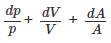(39.6)

Energy Equation

Consider a control volume within the duct shown by dotted lines in Fig. 39.3. The first law of thermodynamics for a control volume fixed in space is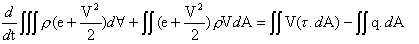(39.7)

where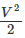is the kinetic energy per unit mass.

Let us discuss the various terms from above equation:

• The first term on the left hand side signifies the rate of change of energy (internal + kinetic) within the control volume
• The second term depicts the flux of energy out of control surface.
• The first term on the right hand side represents the work done on the control surface
• The second term on the right means the heat transferred through the control surface.

It is to be noted that dA is directed along the outward normal.

• Assuming steady state, the first term on the left hand side of Eq. (39.7) is zero. Writing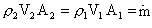(where the subscripts are for Sections 1 and 2), the second term on the left of Eq. (39.7) yields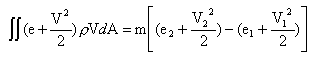The work done on the control surfaces is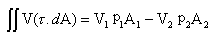The rate of heat transfer to the control volume is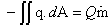where Q is the heat added per unit mass (in J/kg).

• Invoking all the aforesaid relations in Eq. (39.7) and dividing by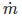, we get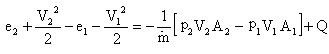(39.8)

We know that the density p is given by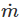/VA hence the first term on the right may be expressed in terms of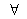(specific volume=1/ρ).
Equation (39.8) can be rewritten as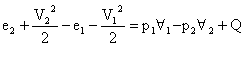(39.9)

• NOTE:-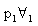is the work done (per unit mass) by the surrounding in pushing fluid into the control volume. Following a similar argument, is the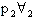work done by the fluid inside the control volume on the surroundings in pushing fluid out of the control volume.

• Since h = e + p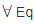. (39.9) gets reduced to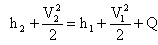(39.10)

This is energy equation, which is valid even in the presence of friction or non-equilibrium conditions between secs 1 and 2.

• It is evident that the sum of enthalpy and kinetic energy remains constant in an adiabatic flow. Enthalpy performs a similar role that internal energy performs in a nonflowing system. The difference between the two types of systems is the flow work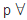required to push the fluid through a section.

Bernoulli and Euler Equations

• For inviscid flows, the steady form of the momentum equation is the Euler equation,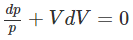(39.11)

Integrating along a streamline, we get the Bernoulli's equation for a compressible flow as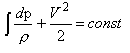(39.12)

• For adiabatic frictionless flows the Bernoulli's equation is identical to the energy equation. Recall, that this is an isentropic flow, so that the Tds equation is given by

Tds = dh - vdp

For isentropic flow, ds=0

Therefore,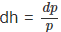Hence, the Euler equation (39.11) can also be written as

Vdv + dh = 0

This is identical to the adiabatic form of the energy Eq. (39.10).

Momentum Principle for a Control Volume

For a finite control volume between Sections 1 and 2 (Fig. 39.3), the momentum principle is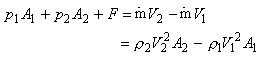(39.13)

where F is the x-component of resultant force exerted on the fluid by the walls. Note that the momentum principle, Eq. (39.13), is applicable even when there are frictional dissipative processes within the control volume

The document Speed of Sound - 2 Notes | Study Additional Documents & Tests for Mechanical Engineering - Mechanical Engineering is a part of the Mechanical Engineering Course Additional Documents & Tests for Mechanical Engineering.
All you need of Mechanical Engineering at this link: Mechanical Engineering

## Additional Documents & Tests for Mechanical Engineering

1 videos|19 docs|45 tests
 Use Code STAYHOME200 and get INR 200 additional OFF

## Additional Documents & Tests for Mechanical Engineering

1 videos|19 docs|45 tests

Track your progress, build streaks, highlight & save important lessons and more!

,

,

,

,

,

,

,

,

,

,

,

,

,

,

,

,

,

,

,

,

,

;